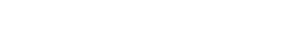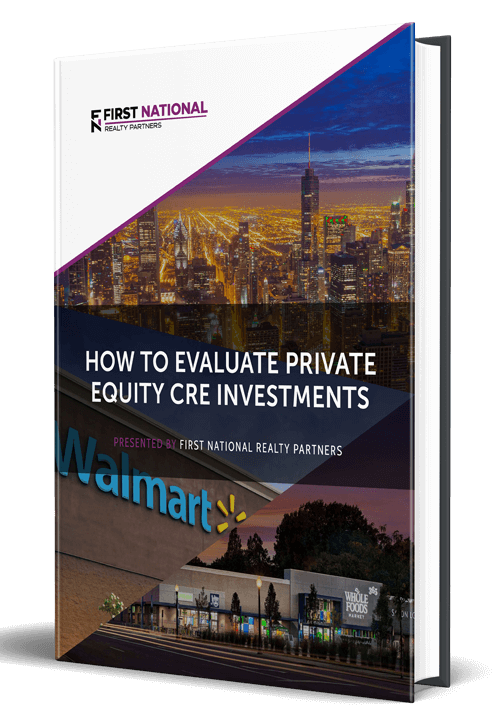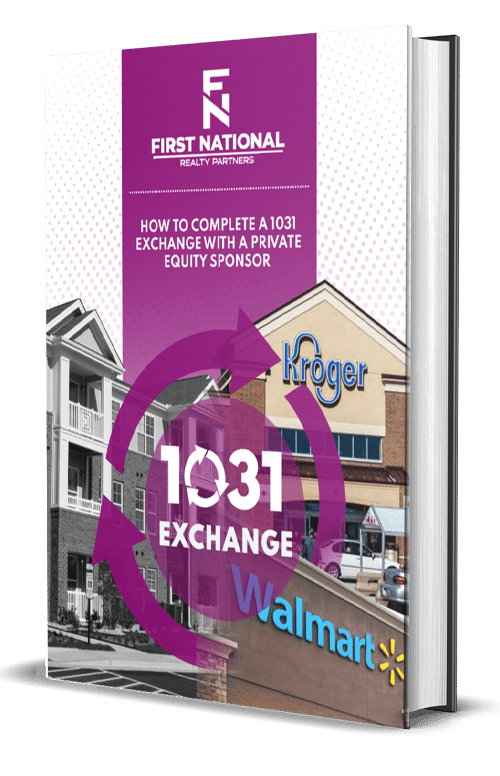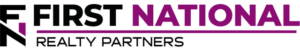# Cap Rate vs. Yield in Commercial Real Estate

### Key Takeaways

• The cap rate is a real estate metric that measures the relationship between a property’s net operating income and its value.  It is calculated as net operating income divided by value.
• Yield is a metric that measures the relationship between a property’s income and its cost.  There are two varieties of yield, levered and unlevered.  The difference between the two is the use of debt.
• The key difference between the cap rate and yield is that cap rate is calculated using a property’s value and yield is calculated using a property’s costAt the time of purchase, these could be the same, but over time they will drift apart.
• When trying to determine what a good cap rate or yield is, there is some subjectivity involved.  Most commercial real estate assets trade in the 4% – 10% cap rate range, while a strong yield is generally in the 8% – 15% range.  But there are certainly exceptions to both ranges depending on the specific characteristics of the property.

When a commercial real estate investor has capital to invest, it can be challenging to figure out which deal(s) to deploy it into.  Often, this means having to filter through dozens or hundreds of real estate investing opportunities to figure out which ones hold the most promise.  In order to do this, investors need to define their “filters,” and one of the ways this can be done is to define their required return criteria.

In this article,  we are going to discuss two of the most important return metrics in commercial real estate (CRE), the cap rate and the yield.  We will define what cap rate and yield are, describe how they are calculated, identify why they are important, and illustrate each of them with examples.  By the end, readers will be able to calculate the cap rate and yield metrics on their own and understand the key differences between them.

At First National Realty Partners, we use both of these real estate metrics as described above.  We use them to filter out the deals that don’t meet our stringent investment criteria, leaving only the most promising opportunities to present to our investors.  To learn more about our current investment opportunities, click here.

## What is the Capitalization Rate (Cap Rate)?

In a real estate investment, the capitalization rate, or cap rate for short, is a metric that describes the relationship between a property’s net operating income (NOI) and its market value.  When calculating cap rate, a few key pieces of information are revealed about a potential investment property:

• Potential Return:  The cap rate represents the potential rate of return on a property assuming that it was purchased with cash.
• Risk:  Because it measures the return, the cap rate can also be used as a proxy for the market’s perceived risk in a property.  A higher cap rate means that the market sees more risk and demands higher returns.  A lower cap rate means that the market sees lower risk, so investors are willing to accept a lower return.  Cap rates tend to vary by location and property type.  For example, the cap rate for an office building in Des Moines is likely to be higher than the cap rate for a multifamily commercial property in Miami.
• Asset Value:  Based on the inputs in the cap rate calculation, it can also be used to calculate a property’s potential value.  This is particularly helpful when trying to estimate purchase and sales prices in a pro forma

Given the points above, it can be seen that the cap rate is an important and versatile metric, so it stands to reason that commercial real estate investors should be familiar with how it is calculated.

### Calculating the Cap Rate

The cap rate formula is NOI divided by property value:

• Cap Rate = Net Operating Income / Property Value

While the cap rate formula itself is straightforward, obtaining the required inputs can be a little bit tricky.

Net operating income (NOI) is calculated as a property’s gross annual income less its operating expenses (property taxes, maintenance, etc).  Property value isn’t always known at the time of calculation, so it could be represented by a value estimate, purchase price, or appraised value.  For example, if a property has an NOI of \$100,000 and a value of \$1,000,000, the resulting cap rate is 10%.

## What is A Property’s Yield?

Yield is a measure of a real estate investor’s annual return based on the amount paid for the property.  The yield’s primary focus is on the return produced by income, not capital appreciation. So, while cap rate measures income divided by price or value, yield measures income divided by total cost.

### Calculating Yield

The formula used to calculate yield is annual income divided by total cost:

• Yield = Annual Income / Total Cost

The yield can be measured on a “levered,” meaning with debt, or an “unlevered,” meaning without debt, basis.  To that end, the formula above can be adjusted to reflect which yield is being calculated:

• Unlevered Yield = Net Operating Income / Total Cost
• Levered Yield = Cash Flow After Debt Service / Down Payment

In most cases, the levered yield will be higher than the unlevered yield because there is less cash put into the deal up front.

## Difference Between Cap Rate and Yield

The key difference between the cap rate and yield is in the denominator of the equations used to calculate these metrics.  The cap rate calculation utilizes the property’s current market value, which changes over time.  The yield calculation utilizes the property’s total cost, which is a static, one time number.

At the time real estate is purchased, the cap rate and the yield may be similar.  But, as the market value of the property changes over time, the yield and cap rate metrics will drift apart.

## How Does Cap Rate Affect Yield?

Both the cap rate and the yield are measures of annual returns.  But, remember, the cap rate uses the property value as a denominator in the formula.  As property values rise, cap rates fall.  For example, if a property produces \$100,000 in NOI and has a value of \$1,000,000, the resulting cap rate is 10%.  If that same \$100,000 in NOI is applied to a \$1,200,000 property, the resulting cap rate is 8.3%.

So, with respect to the yield, rising property prices/falling cap rates could result in falling yields.  This is because the property would become more expensive to purchase.  As the property becomes more expensive, the yield falls.

## Cap Rate vs. Yield Example

To illustrate how the cap rate and yield work in real estate investing, an example is helpful.  Suppose that an investor is considering the purchase of a property with the following characteristics:

• Purchase Price:  \$5,000,000
• Market Value:  \$5,250,000
• Net Operating Income:  \$400,000
• Cash Flow After Debt Service:  \$200,000
• Down Payment:  \$1,000,000

Based on this information, the following metrics can be calculated:

• Cap Rate:  \$400,000 / \$5,250,000 = 7.61%
• Unlevered Yield:  \$400,000 / \$5,000,000 = 8.00%
• Levered Yield: \$200,000 / \$1,000,000 = 20.00%

It should be noted that there is a big difference between the property’s purchase price and the market value.  This is not necessarily the norm, but this was the case for illustrative purposes.  That difference also results in a difference between the cap rate and yield.  In this scenario, the cap rate is 7.61% and the unlevered yield is 8.00%.  But, when debt is added into the equation, the levered yield rises to 20%.

## What is a Good Cap Rate and What Is A Good Yield?

Cap rates are somewhat subjective, which means there is no objectively “good” cap rate.  Cap rates are highly dependent on the market, property type, stability of rental income, growth rate, leasing activity, and condition of the property.  Most commercial properties trade in a general cap rate range of 4% – 10%, but there are certainly exceptions to this range based on the characteristics described above.

Yield is a bit more objective than cap rate, but it is still measured relative to a property investor’s return requirements.  Generally, a yield in the 8% – 15% range is considered good.  But, if a real estate investor requires a 20% return, a 15% yield doesn’t seem as good.

## Summary & Conclusion

• The cap rate is a real estate metric that measures the relationship between a property’s net operating income and its value.  It is calculated as net operating income divided by value.
• Yield is a real estate metric that measures the relationship between a property’s income and its cost.  There are two varieties of yield, levered and unlevered.  The difference between the two is the use of debt.
• The key difference between the cap rate and yield is that cap rate is calculated using a property’s value and yield is calculated using a property’s costAt the time of purchase, cap rate and yield could be the same, but over time they will drift apart.
• When trying to determine what a good cap rate or yield is, there is some subjectivity involved.  Most commercial real estate assets trade in the 4% – 10% cap rate range, while a strong yield is generally in the 8% – 15% range.  But there are certainly exceptions to both ranges depending on the specific characteristics of the property.

## Interested In Learning More?

First National Realty Partners is one of the country’s leading private equity commercial real estate investment firms. With an intentional focus on finding world-class, multi-tenanted assets well below intrinsic value, we seek to create superior long-term, risk-adjusted returns for our investors while creating strong economic assets for the communities we invest in.

Get instant access to all of our current and past commercial real estate deals.## Subscribe Now

Get the latest news on real estate

FNRP Spotlight Series

1031 Exchange

Acquisitions

## Get More From FNRP## How to Evaluate Private Equity CRE Investments## How to Complete a 1031 Exchange with a Private Equity Sponsor